# How to Calculate and Solve for Dryer Capacity | Polymer & Textile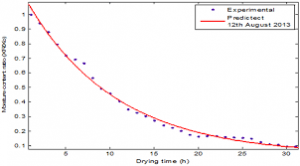The image above represents dryer capacity.

To compute for dryer capacity, two essential parameters are needed and these parameters are material consumption (Mcand drying time (TD).

The formula for calculating dryer capacity:

Dc = Mc / TD

Where:

Dc = Dryer Capacity
Mc = Material Consumption
TD = Drying Time

Let’s solve an example;
Given that the material consumption is 28 and the drying time is 34. Find the dryer capacity?

This implies that;

Mc = Material Consumption = 28
TD = Drying Time = 34

Dc = Mc / TD
Dc = 28 / 34
Dc = 0.82

Therefore, the dryer capacity is 0.82.

Calculating for Material Consumption when the Dryer Capacity and the Drying time is Given.

Mc = Dc x TD

Where:

Mc = Material Consumption
Dc = Dryer Capacity
TD = Drying Time

Let’s solve an example;
Find the material consumption when the dryer capacity is 40 and the drying time is 2.

This implies that;

Dc = Dryer Capacity = 40
TD = Drying Time = 2

Mc = Dc x TD
Mc = 40 x 2
Mc = 80

Therefore, the material consumption is 80.

Calculating for Drying time when the Dryer Capacity and the Material Consumption is Given.

TD = Mc / Dc

Where:

TD = Drying Time
Dc = Dryer Capacity
Mc = Material Consumption

Let’s solve an example;
Find the drying time of a given dryer capacity of 38 and a material consumption of 18.

This implies that;

Dc = Dryer Capacity = 38
Mc = Material Consumption = 18

TD = Mc / Dc
TD = 18 / 38
TD = 0.47

Therefore, the drying time is 0.47.

Nickzom Calculator – The Calculator Encyclopedia is capable of calculating the dryer capacity.

To get the answer and workings of the dryer capacity using the Nickzom Calculator – The Calculator Encyclopedia. First, you need to obtain the app.

You can get this app via any of these means:

To get access to the professional version via web, you need to register and subscribe for NGN 1,500 per annum to have utter access to all functionalities.
You can also try the demo version via https://www.nickzom.org/calculator

Apple (Paid) – https://itunes.apple.com/us/app/nickzom-calculator/id1331162702?mt=8
Once, you have obtained the calculator encyclopedia app, proceed to the Calculator Map, then click on Polymer & Textile under Engineering.Now, Click on Dryer Capacity under Polymer & Textile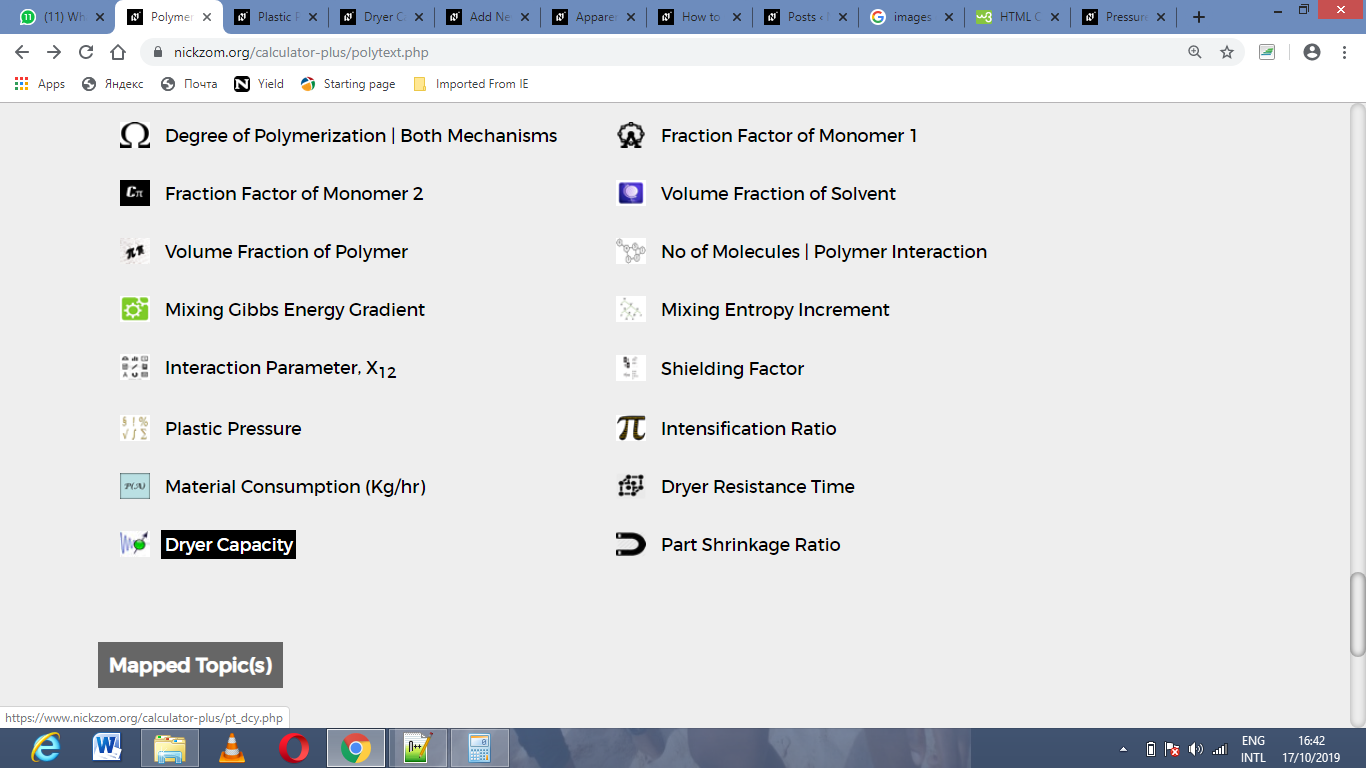The screenshot below displays the page or activity to enter your values, to get the answer for the dryer capacity according to the respective parameters which is the material consumption (Mcand drying time (TD).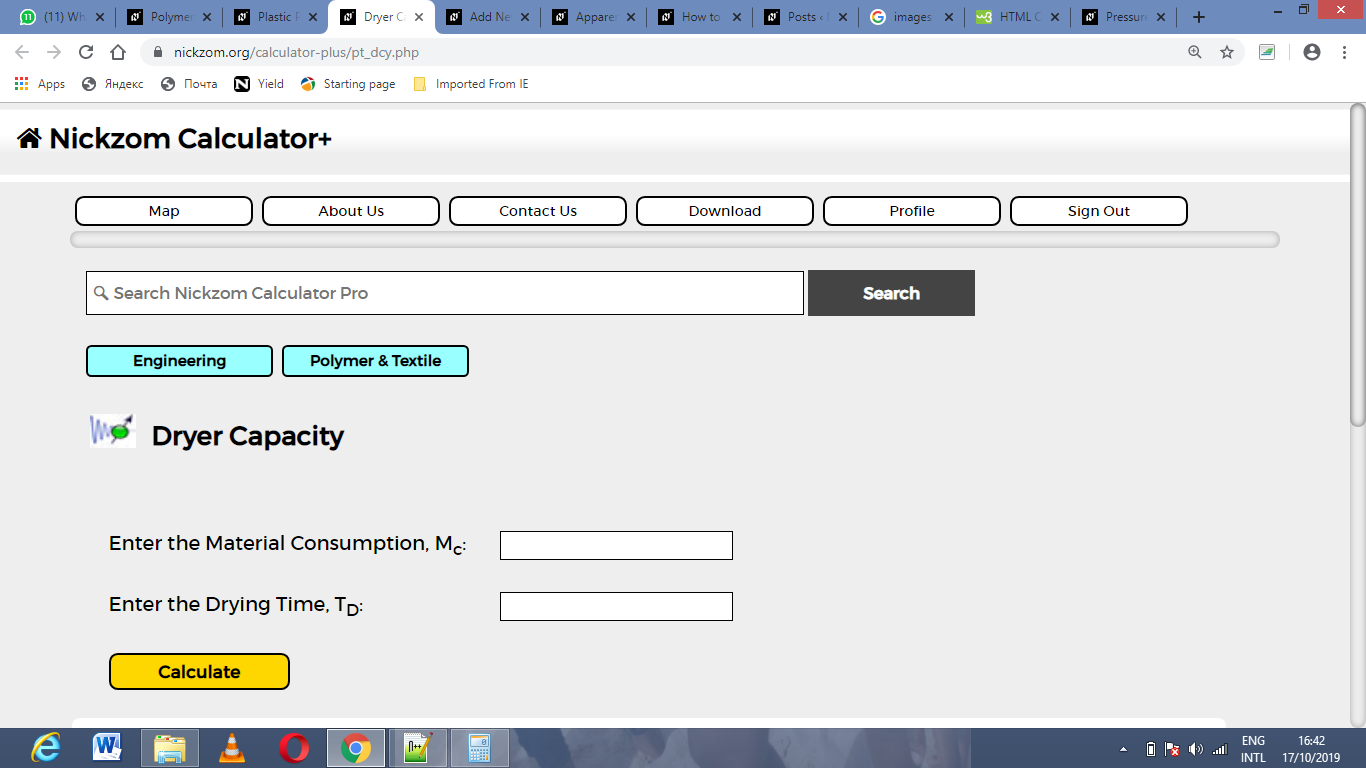Now, enter the values appropriately and accordingly for the parameters as required by the material consumption (Mc) is 28 and drying time (TD) is 34.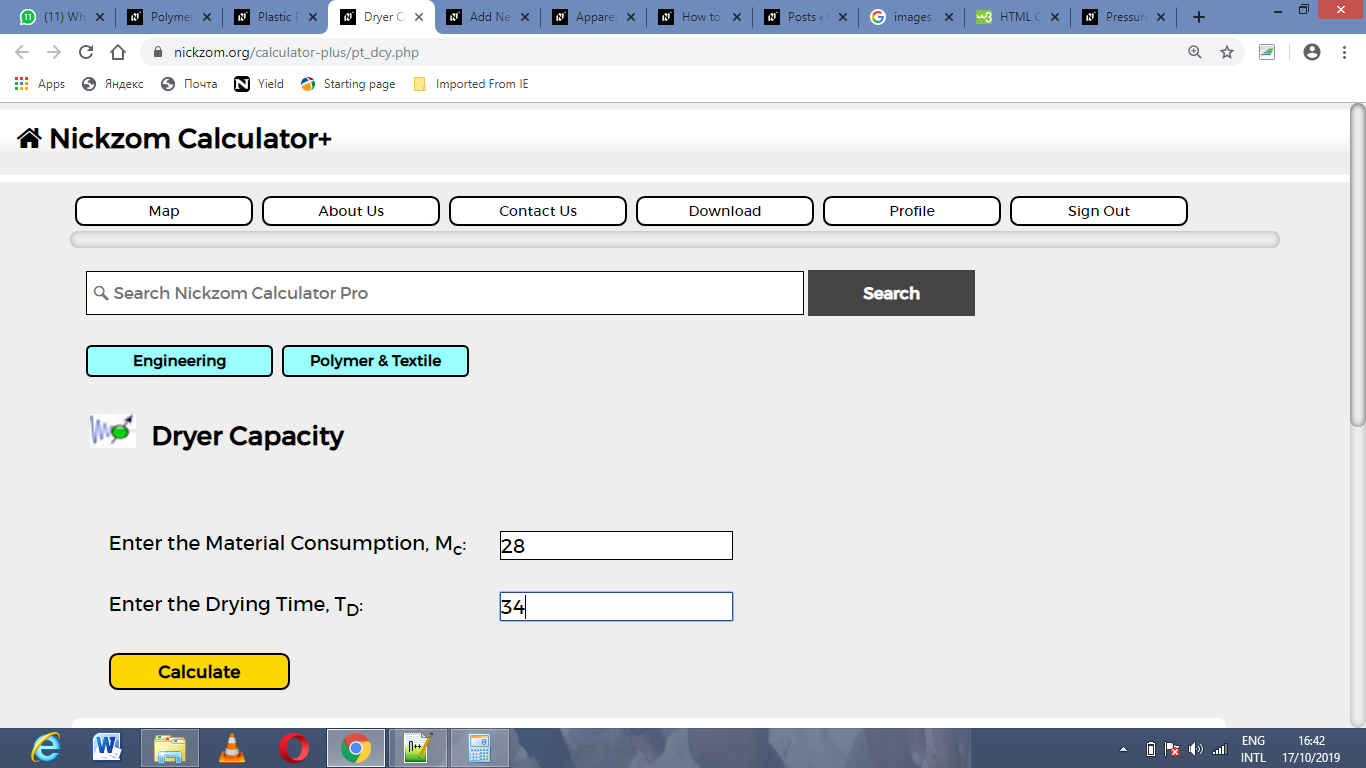Finally, Click on Calculate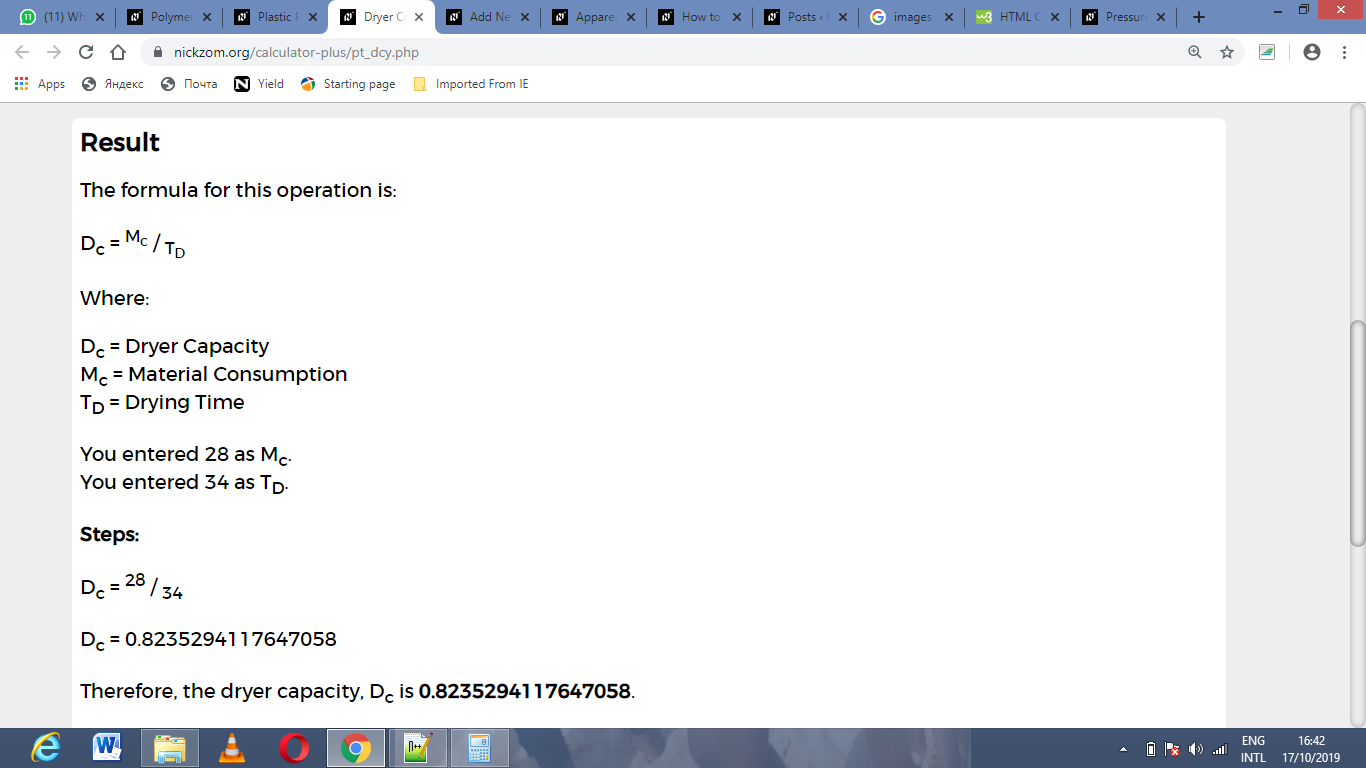As you can see from the screenshot above, Nickzom Calculator– The Calculator Encyclopedia solves for the dryer capacity and presents the formula, workings and steps too.